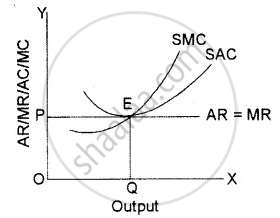# Answer the Following Question. Show with the Help of a Diagram, How a Perfectly Competitive Firm Earns Normal Profit in Short-run Equilibrium. - Economics

Short Note

Show with the help of a diagram, how a perfectly competitive firm earns normal profit in short-run equilibrium.

#### Solution

Under perfect competition a firm’s, average revenue curve is equal to the marginal revenue curve due to uniform prices for homogeneous goods. In, short-run, a competitive firm can earn supernormal profits, normal profits can suffer losses also. The adjacent diagram shows a perfectly competitive firm earning normal profits. Since the firm is a price taker, it has to decide the amount of output it should produce at the given price so as to maximize the profits following the equilibrium conditions.
SMC = MR and AR = ACIn the given diagram OQ is equilibrium output where SMC is equal to MR Since SAC is tangent to P = AR = MR line, the firm covers only its SAC which includes normal profits.

Concept: Features of Perfect Competition
Is there an error in this question or solution?
2014-2015 (March) Set 1

Share